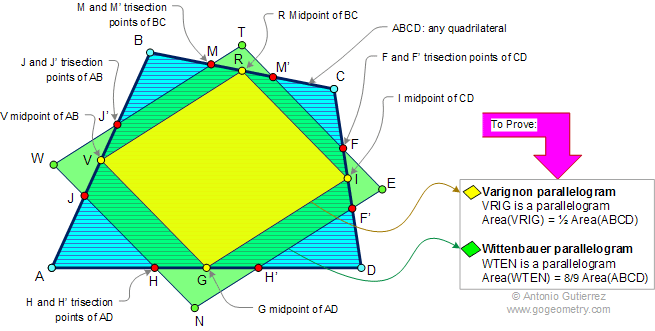Varignon and Wittenbauer Parallelograms, Area Measurement. Level: High School, SAT Prep, College geometry. Varignon parallelogram. The figure formed when the midpoints of the sides of any quadrilateral are joined in order is a parallelogram. Its area is one half that of the quadrilateral. Wittenbauer parallelogram. If the trisectors of each side of a quadrilateral are found, the figure formed by connecting and extending adjacent points on either side of a vertex is a parallelogram. Its area is 8/9 of the quadrilateral.Recent Additions